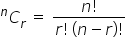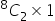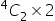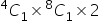Maths-
General
Easy

Question

# The greatest possible number of points of intersections of 8 straight line and 4 circles is :

## 32    64    76    104Hint:

## The correct answer is: 104

### Complete step-by-step answer:The number point of intersection between two circles can be counted by finding the number of ways in which one circle and one line can be selected out of the lot multiplied by 2 as one circle and one line can intersect at most two points.For selecting r objects from n objects can be done by using the formula as followsAs mentioned in the question, we have to find the total number of intersection points.For calculating the points of intersection between two lines, we can use the formula which is mentioned in the hint as follows == 28 For calculating the points of intersection between two circles, we can use the formula which is mentioned in the hint as follows == 12For calculating the points of intersection between one line and one circle, we can use the formula which is mentioned in the hint as follows= 64Hence, the total number of points of intersection is = 28 + 12  + 64 = 104

The students can make an error if they don’t know about the formula for calculating the number of points as mentioned in the hint which is as follows
The number point of intersection between two lines can be counted by finding the number of ways in which two lines can be selected out of the lot as two lines can intersect at most one point.
The number point of intersection between two circles can be counted by finding the number of ways in which two circles can be selected out of the lot multiplied by 2 as two circles can intersect at most two points.
The number point of intersection between two circles can be counted by finding the number of ways in which one circle and one line can be selected out of the lot multiplied by 2 as one circle and one line can intersect at most two points.

### Related Questions to study#### With Turito Foundation.#### Get an Expert Advice From Turito.